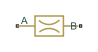# Fixed Orifice

(To be removed) Hydraulic orifice with constant cross-sectional area

The Hydraulics (Isothermal) library will be removed in a future release. Use the Isothermal Liquid library instead. (since R2020a)

•Libraries:
Simscape / Fluids / Hydraulics (Isothermal) / Orifices

## Description

The Fixed Orifice block models a sharp-edged constant-area orifice, flow rate through which is proportional to the pressure differential across the orifice. The flow rate is determined according to the following equations:

`$q={C}_{D}\cdot A\sqrt{\frac{2}{\rho }}\cdot \frac{p}{{\left({p}^{2}+{p}_{cr}^{2}\right)}^{1/4}}$`
`$\Delta p={p}_{\text{A}}-{p}_{\text{B}},$`

where

 q Flow rate p Pressure differential pA, pB Gauge pressures at the block terminals CD Flow discharge coefficient A Orifice passage area ρ Fluid density pcr Minimum pressure for turbulent flow

The minimum pressure for turbulent flow, pcr, is calculated according to the laminar transition specification method:

• By pressure ratio — The transition from laminar to turbulent regime is defined by the following equations:

`$\begin{array}{l}{p}_{cr}=\left({p}_{\text{avg}}+{p}_{atm}\right)\left(2-{B}_{lam}\right)\\ {p}_{\text{avg}}=\left({p}_{\text{A}}+{p}_{\text{B}}\right)/2\end{array}$`

where

 pavg Average pressure between the block terminals patm Atmospheric pressure, 101325 Pa Blam Pressure ratio at the transition between laminar and turbulent regimes (Laminar flow pressure ratio parameter value)
• By Reynolds number — The transition from laminar to turbulent regime is defined by the following equations:

`${p}_{cr}=\frac{\rho }{2}{\left(\frac{{\mathrm{Re}}_{cr}\cdot \nu }{{C}_{D}\cdot {D}_{H}}\right)}^{2}$`
`${D}_{H}=\sqrt{\frac{4A}{\pi }}$`

where

 DH Orifice hydraulic diameter ν Fluid kinematic viscosity Recr Critical Reynolds number (Critical Reynolds number parameter value)

The block positive direction is from port A to port B. This means that the flow rate is positive if it flows from A to B, and the pressure differential is determined as $\Delta p={p}_{\text{A}}-{p}_{\text{B}},$.

### Variables

To set the priority and initial target values for the block variables prior to simulation, use the Initial Targets section in the block dialog box or Property Inspector. For more information, see Set Priority and Initial Target for Block Variables.

Nominal values provide a way to specify the expected magnitude of a variable in a model. Using system scaling based on nominal values increases the simulation robustness. Nominal values can come from different sources, one of which is the Nominal Values section in the block dialog box or Property Inspector. For more information, see Modify Nominal Values for a Block Variable.

## Assumptions and Limitations

• Fluid inertia is not taken into account.

## Ports

### Conserving

expand all

Hydraulic conserving port associated with the orifice inlet.

Hydraulic conserving port associated with the orifice outlet.

## Parameters

expand all

Orifice passage area.

Semi-empirical parameter for orifice capacity characterization. Its value depends on the geometrical properties of the orifice, and usually is provided in textbooks or manufacturer data sheets.

Select how the block transitions between the laminar and turbulent regimes:

• `Pressure ratio` — The transition from laminar to turbulent regime is smooth and depends on the value of the Laminar flow pressure ratio parameter. This method provides better simulation robustness.

• `Reynolds number` — The transition from laminar to turbulent regime is assumed to take place when the Reynolds number reaches the value specified by the Critical Reynolds number parameter.

Pressure ratio at which the flow transitions between laminar and turbulent regimes.

#### Dependencies

To enable this parameter, set Laminar transition specification to `Pressure ratio`.

The maximum Reynolds number for laminar flow. The value of the parameter depends on the orifice geometrical profile. You can find recommendations on the parameter value in hydraulics textbooks. The default value is `12`, which corresponds to a round orifice in thin material with sharp edges.

#### Dependencies

To enable this parameter, set Laminar transition specification to `Reynolds number`.

## Version History

Introduced in R2006a

expand all